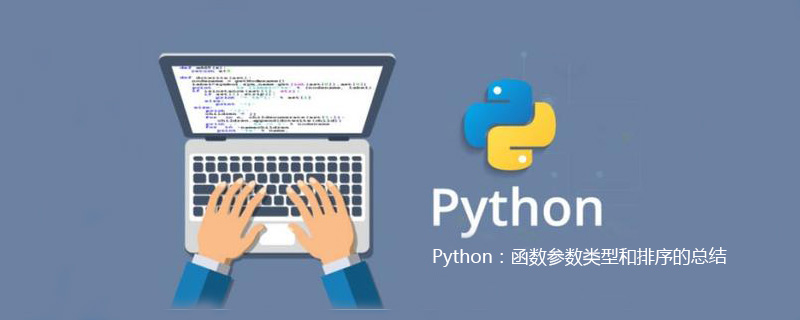Python：函数参数类型和排序的总结Python中函数的参数问题有点复杂，主要是因为参数类型问题导致的情况比较多，下面来分析一下。

1：不存在缺省函数时：

def test(a,*args,c,d=3,**kwargs):
print(a)
print(args)
print(c)
print(d)
print(kwargs)
test(1,2,3,4,c=1,d=4,e=11,f=22)

1
(2, 3, 4)
1
4
{'e': 11, 'f': 22}

2：存在缺省参数，参数取默认值，（缺省参数放在args后面）*

def test(a,*args,b=1,c,d=3,**kwargs):
print(a)
print(args)
print(b)
print(c)
print(d)
print(kwargs)
test(1,2,3,4,c=1,d=4,e=11,f=22,h=66)

(2, 3, 4)
1
{'e': 11, 'f': 22, 'h': 66}

3：存在缺省参数，参数不取默认值，（缺省参数放在args前面）*

def test(a,b=1,*args,c,d=3,**kwargs):
print(a)
print(b)
print(args)
print(c)
print(d)
print(kwargs)
test(1,2,3,4,c=1,d=4,e=11,f=22,h=66)

1
2
(3, 4)
1
4
{'f': 22, 'e': 11, 'h': 66}

Python开发开发项目